# RD Sharma Solutions for Class 8 Maths Chapter 3 - Squares and Square Roots Exercise 3.7

Students can easily download the RD Sharma Solutions for the Exercise 3.7 of Class 8 Maths Chapter 3, Squares and Square Roots from the link accessible here. BYJU’S experts have interpreted the questions from Exercise 3.7 in Maths, and this will help students in solving this exercise. The main intention of our subject experts is to offer better clarity about the concepts among students. The answers are expressed elaborately with steps based on the CBSE syllabus evaluation process. Exercise 3.7 of Class 8 is about finding square roots of rational numbers in decimal form. By practising the RD Sharma Class 8 Solutions students attain good score in their exams.

## Download the pdf of RD Sharma Solutions For Class 8 Maths Chapter 3 Squares and Square Roots Exercise 3.7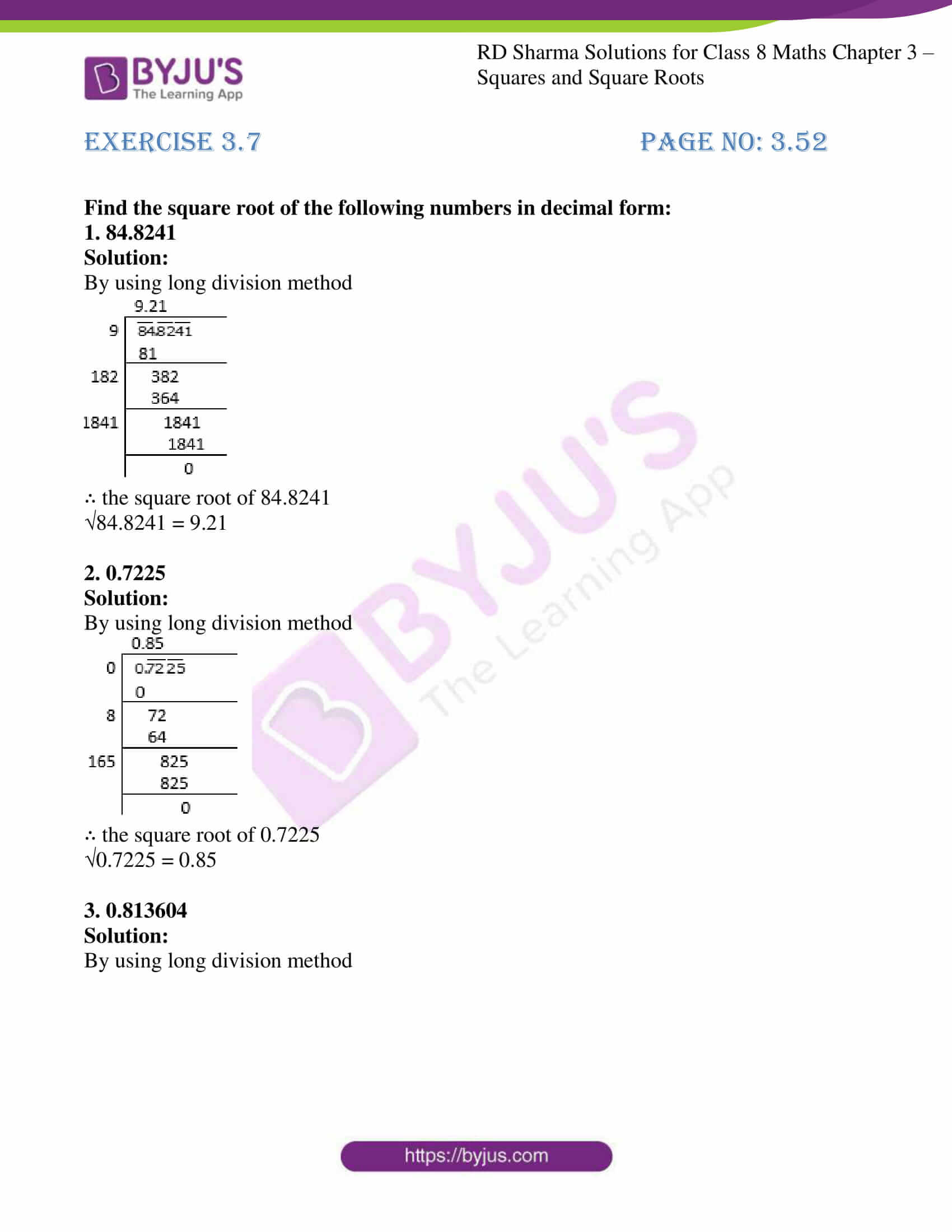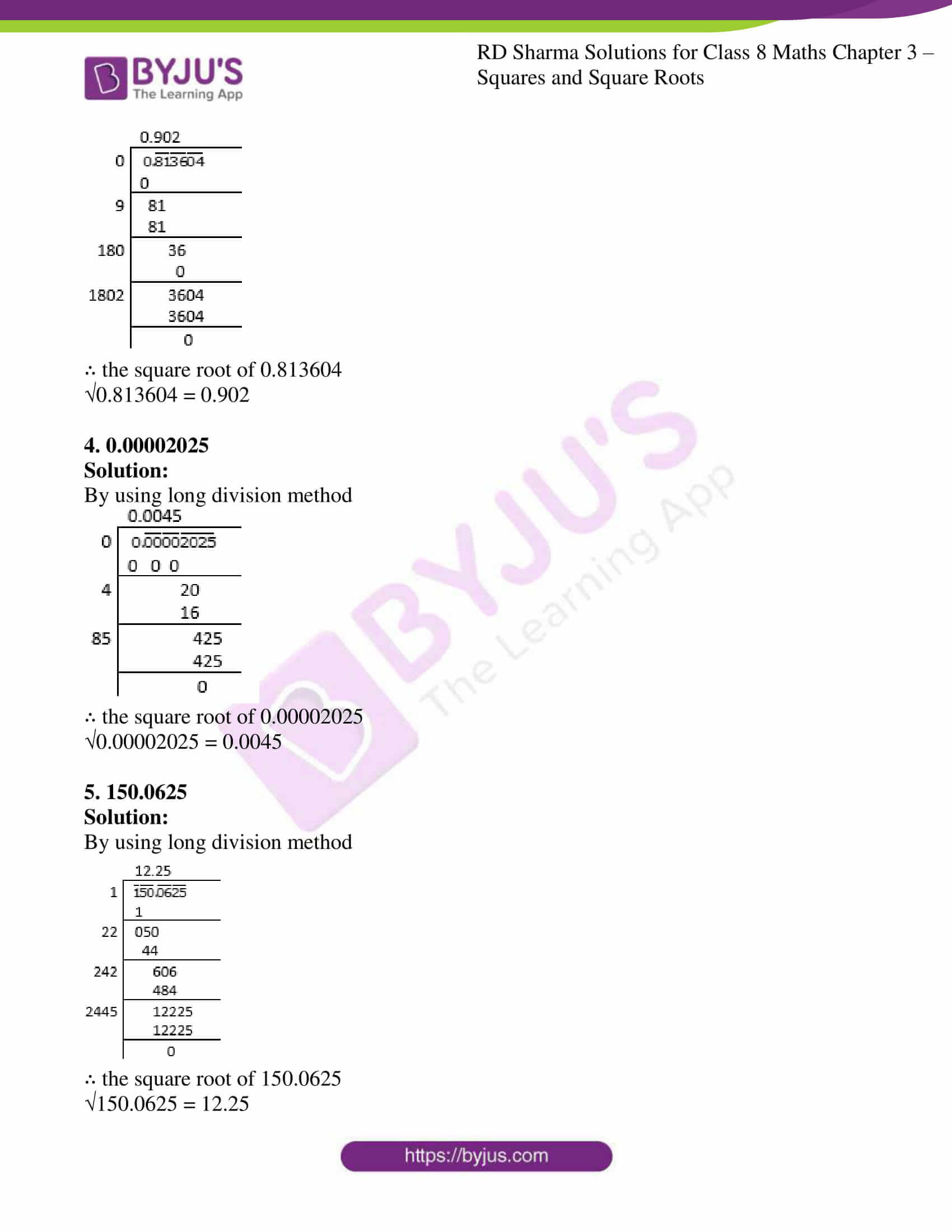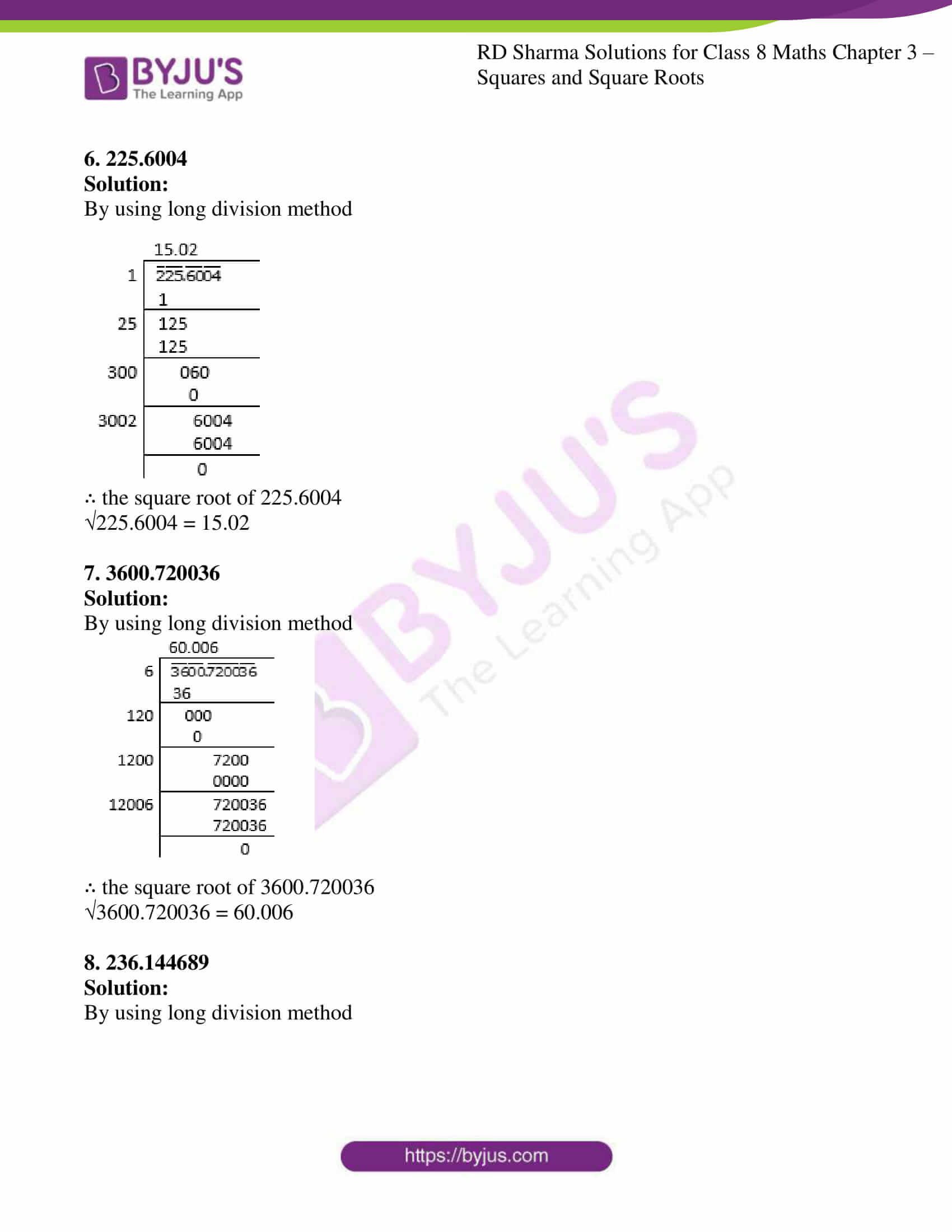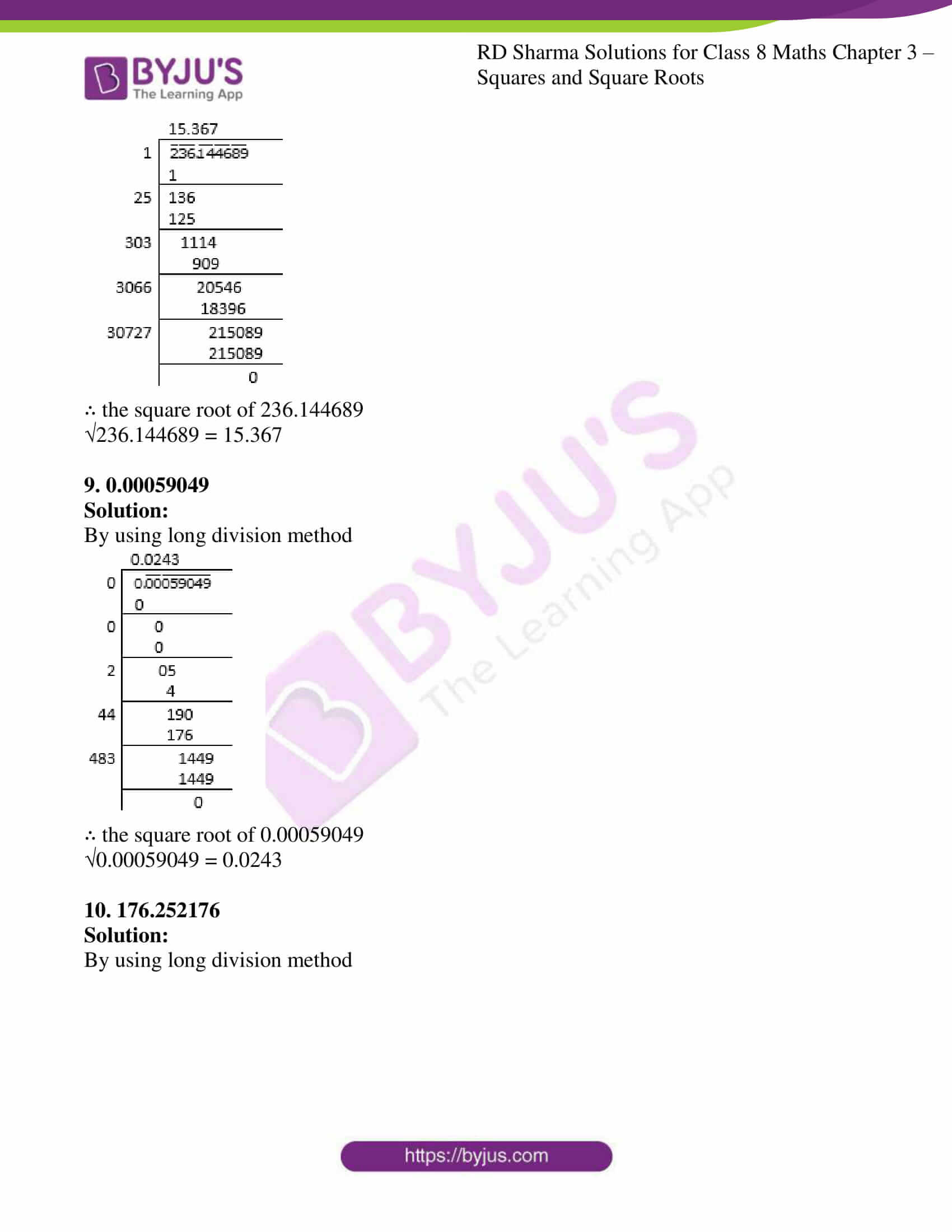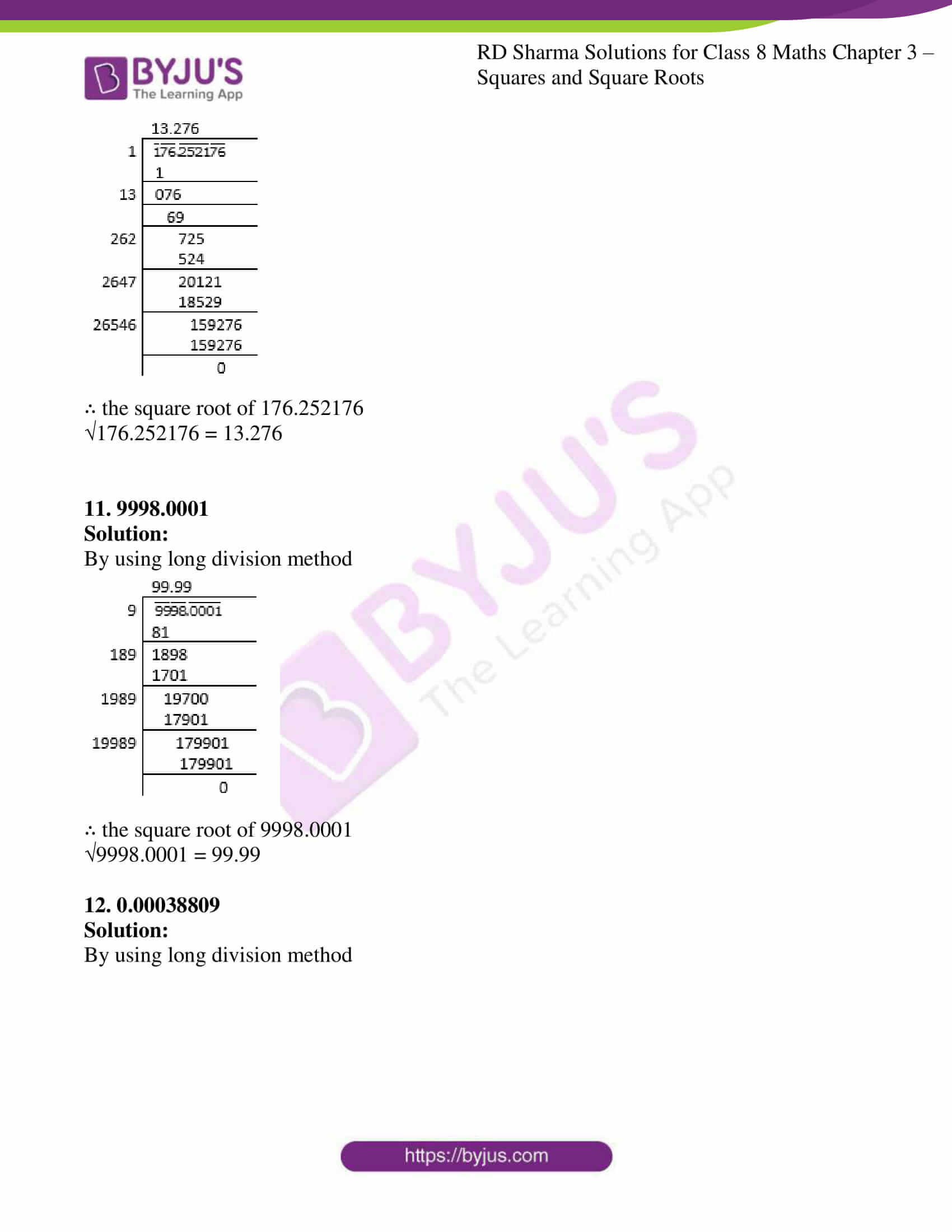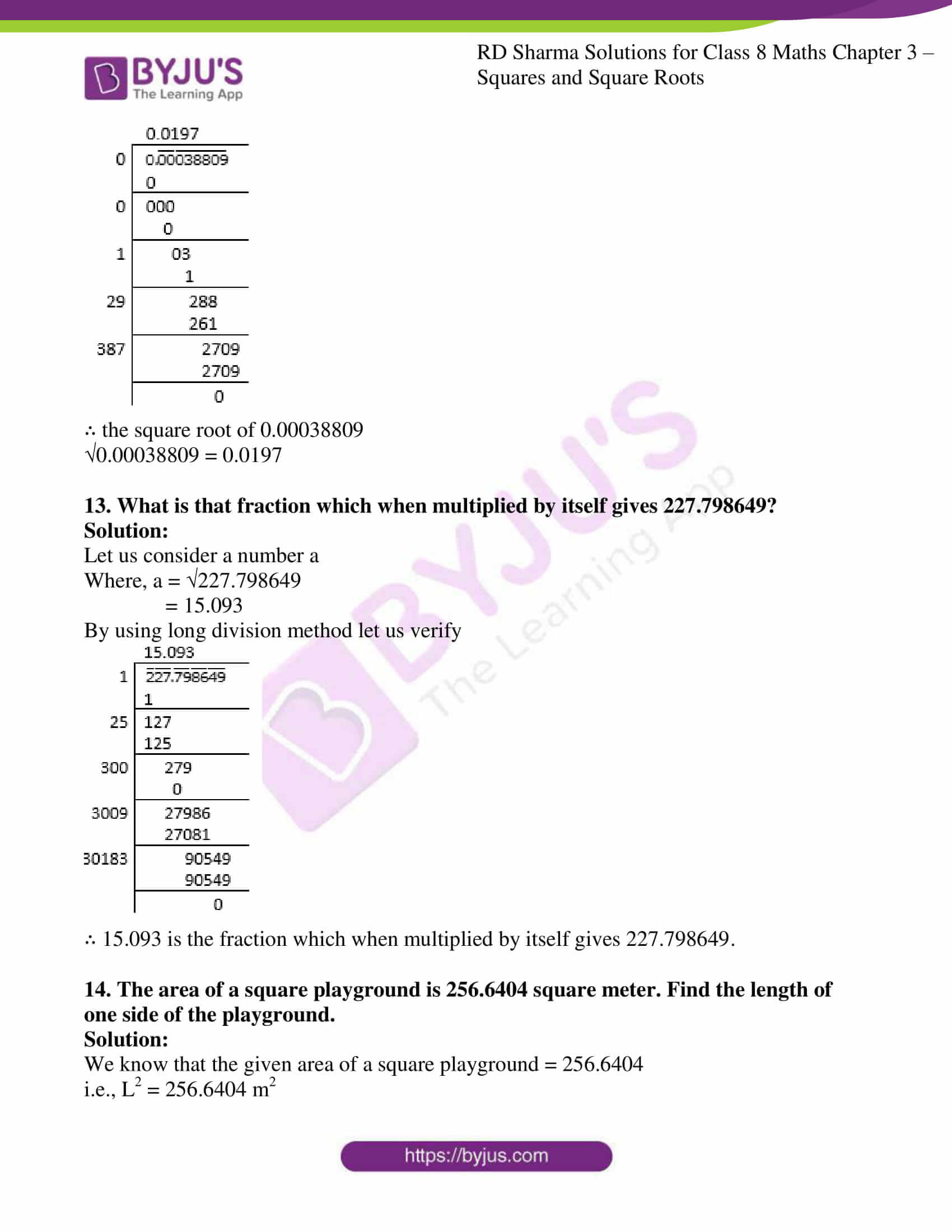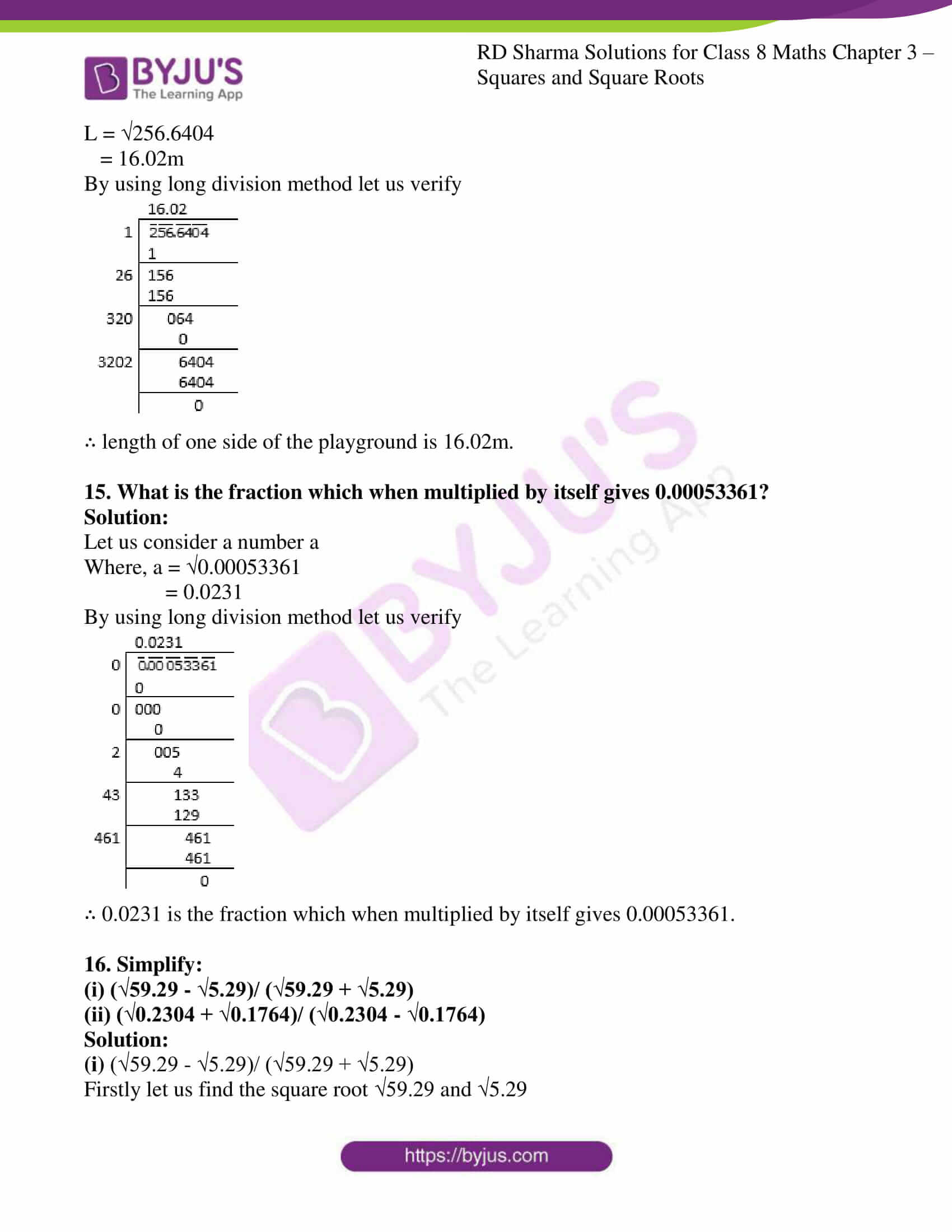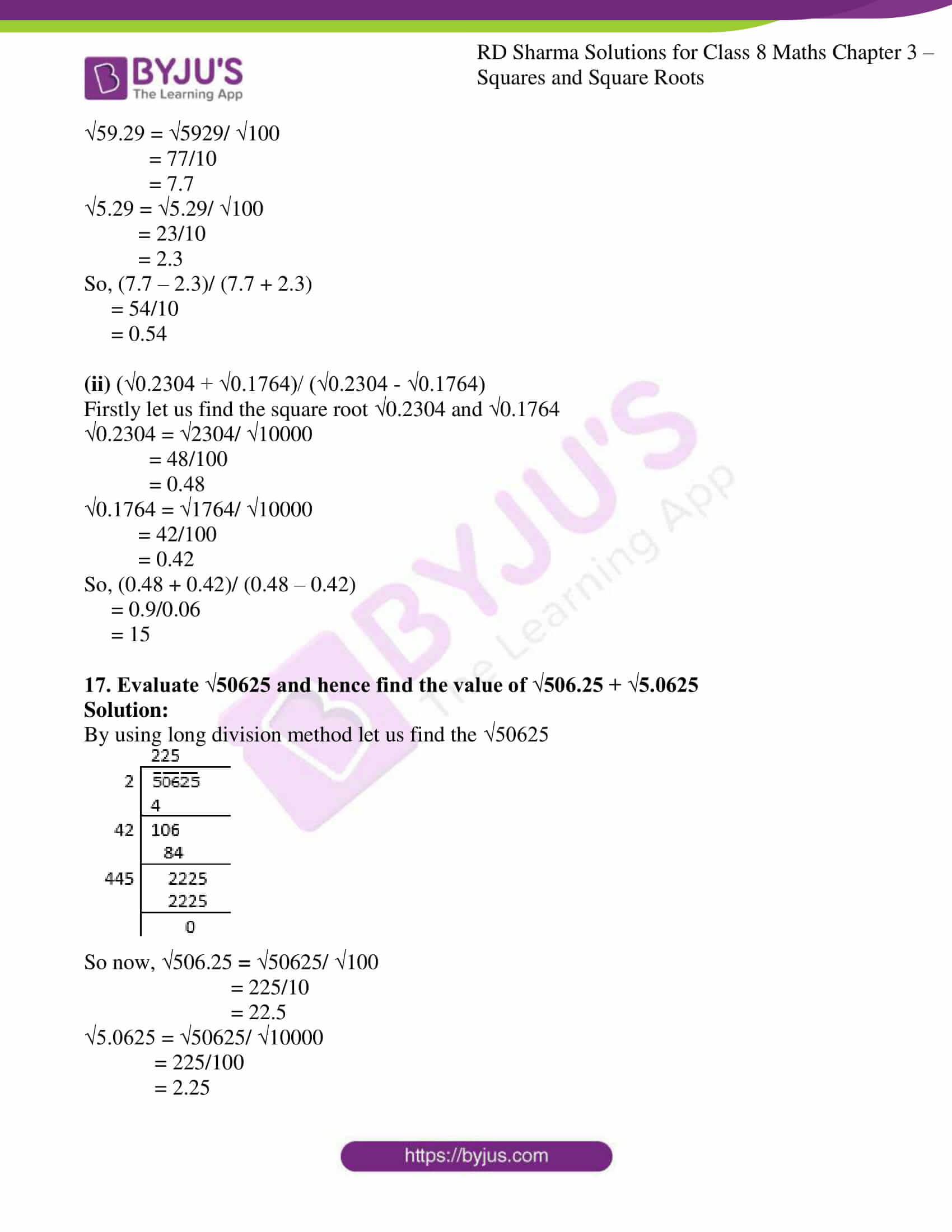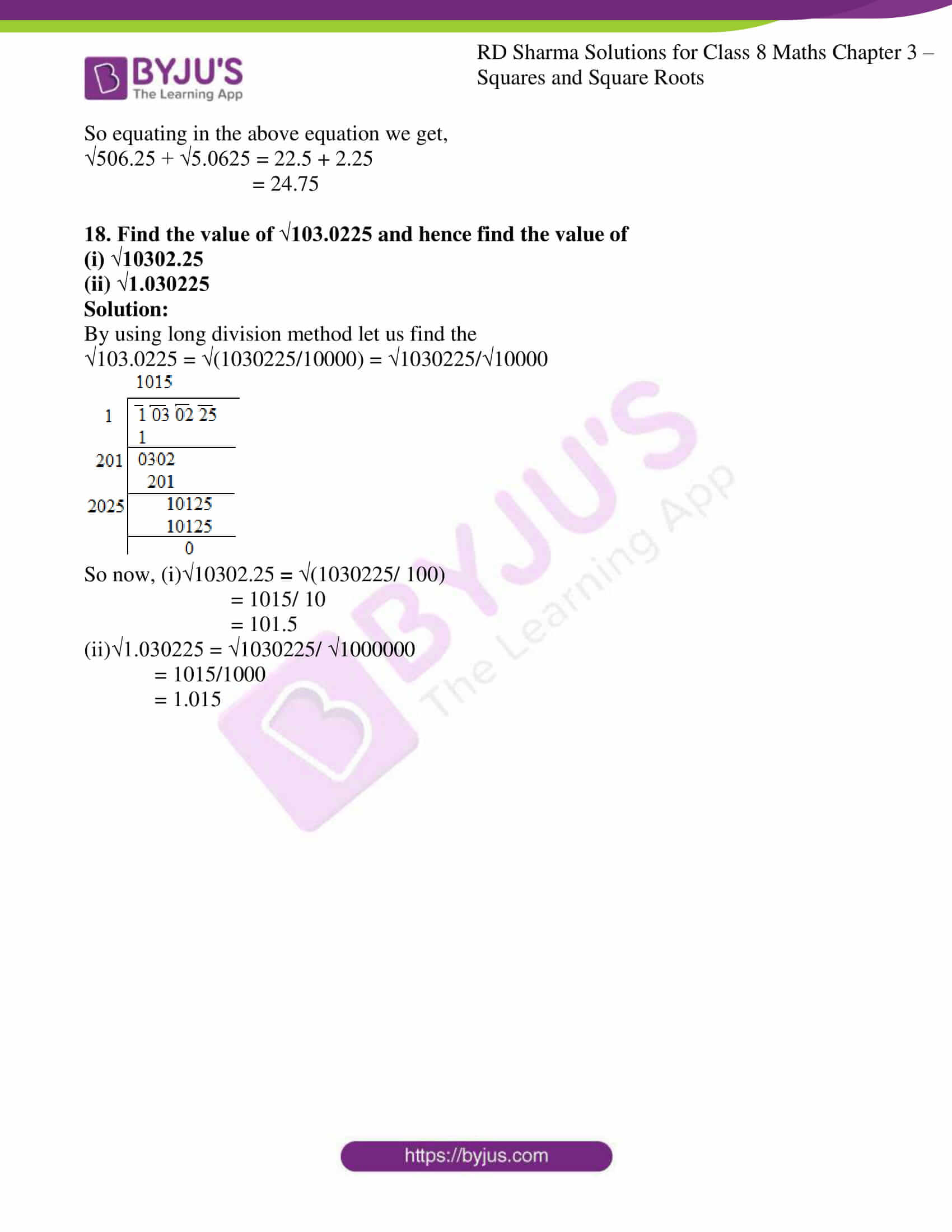### Access Answers to RD Sharma Solutions for Class 8 Maths Exercise 3.7 Chapter 3 Squares and Square Roots

#### EXERCISE 3.7 PAGE NO: 3.52

Find the square root of the following numbers in decimal form:
1. 84.8241

Solution:

By using long division method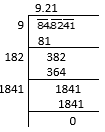∴ the square root of 84.8241

√84.8241 = 9.21

2. 0.7225

Solution:

By using long division method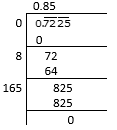∴ the square root of 0.7225

√0.7225 = 0.85

3. 0.813604

Solution:

By using long division method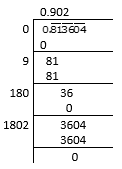∴ the square root of 0.813604

√0.813604 = 0.902

4. 0.00002025

Solution:

By using long division method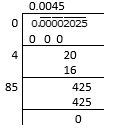∴ the square root of 0.00002025

√0.00002025 = 0.0045

5. 150.0625

Solution:

By using long division method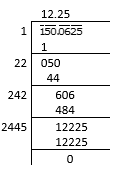∴ the square root of 150.0625

√150.0625 = 12.25

6. 225.6004

Solution:

By using long division method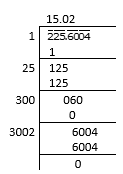∴ the square root of 225.6004

√225.6004 = 15.02

7. 3600.720036

Solution:

By using long division method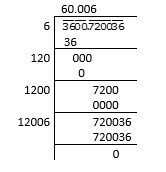∴ the square root of 3600.720036

√3600.720036 = 60.006

8. 236.144689

Solution:

By using long division method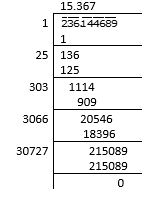∴ the square root of 236.144689

√236.144689 = 15.367

9. 0.00059049

Solution:

By using long division method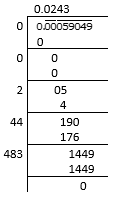∴ the square root of 0.00059049

√0.00059049 = 0.0243

10. 176.252176

Solution:

By using long division method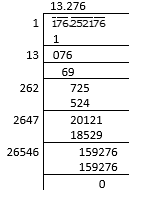∴ the square root of 176.252176

√176.252176 = 13.276

11. 9998.0001

Solution:

By using long division method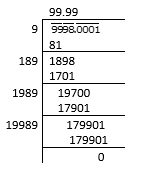∴ the square root of 9998.0001

√9998.0001 = 99.99

12. 0.00038809

Solution:

By using long division method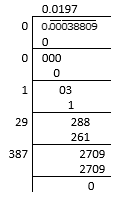∴ the square root of 0.00038809

√0.00038809 = 0.0197

13. What is that fraction which when multiplied by itself gives 227.798649?

Solution:

Let us consider a number a

Where, a = √227.798649

= 15.093

By using long division method let us verify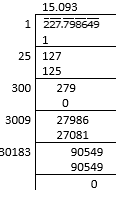∴ 15.093 is the fraction which when multiplied by itself gives 227.798649.

14. The area of a square playground is 256.6404 square meter. Find the length of one side of the playground.

Solution:

We know that the given area of a square playground = 256.6404

i.e., L2 = 256.6404 m2

L = √256.6404

= 16.02m

By using long division method let us verify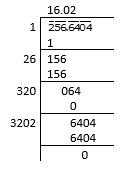∴ length of one side of the playground is 16.02m.

15. What is the fraction which when multiplied by itself gives 0.00053361?

Solution:

Let us consider a number a

Where, a = √0.00053361

= 0.0231

By using long division method let us verify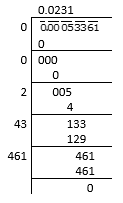∴ 0.0231 is the fraction which when multiplied by itself gives 0.00053361.

16. Simplify:

(i) (√59.29 – √5.29)/ (√59.29 + √5.29)

(ii) (√0.2304 + √0.1764)/ (√0.2304 – √0.1764)

Solution:

(i) (√59.29 – √5.29)/ (√59.29 + √5.29)

Firstly let us find the square root √59.29 and √5.29

√59.29 = √5929/ √100

= 77/10

= 7.7

√5.29 = √5.29/ √100

= 23/10

= 2.3

So, (7.7 – 2.3)/ (7.7 + 2.3)

= 54/10

= 0.54

(ii) (√0.2304 + √0.1764)/ (√0.2304 – √0.1764)

Firstly let us find the square root √0.2304 and √0.1764

√0.2304 = √2304/ √10000

= 48/100

= 0.48

√0.1764 = √1764/ √10000

= 42/100

= 0.42

So, (0.48 + 0.42)/ (0.48 – 0.42)

= 0.9/0.06

= 15

17. Evaluate √50625 and hence find the value of √506.25 + √5.0625

Solution:

By using long division method let us find the √50625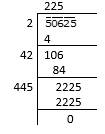So now, 506.25 = √50625/ √100

= 225/10

= 22.5

√5.0625 = √50625/ √10000

= 225/100

= 2.25

So equating in the above equation we get,

√506.25 + √5.0625 = 22.5 + 2.25

= 24.75

18. Find the value of √103.0225 and hence find the value of
(i) √10302.25
(ii) √1.030225

Solution:

By using long division method let us find the

√103.0225 = √(1030225/10000) = √1030225/√10000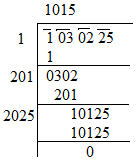So now, (i)10302.25 = √(1030225/ 100)

= 1015/ 10

= 101.5

(ii)√1.030225 = √1030225/ √1000000

= 1015/1000

= 1.015

## RD Sharma Solutions for Class 8 Maths Exercise 3.7 Chapter 3 – Squares and Square Roots

Exercise 3.7 of RD Sharma Solutions for Chapter 3 Squares and Square Roots, this exercise mainly deals with the concepts related to the square roots of rational numbers in decimal form. RD Sharma Solutions can help the students in practising and learning each and every concept as it provides solutions to all questions asked in the RD Sharma textbook. Very lengthy questions that require many steps can be easily remembered by the students by using RD Sharma Solutions. Those who aim to secure high marks in Maths of Class 8 are advised to practice all the questions present in the textbook.